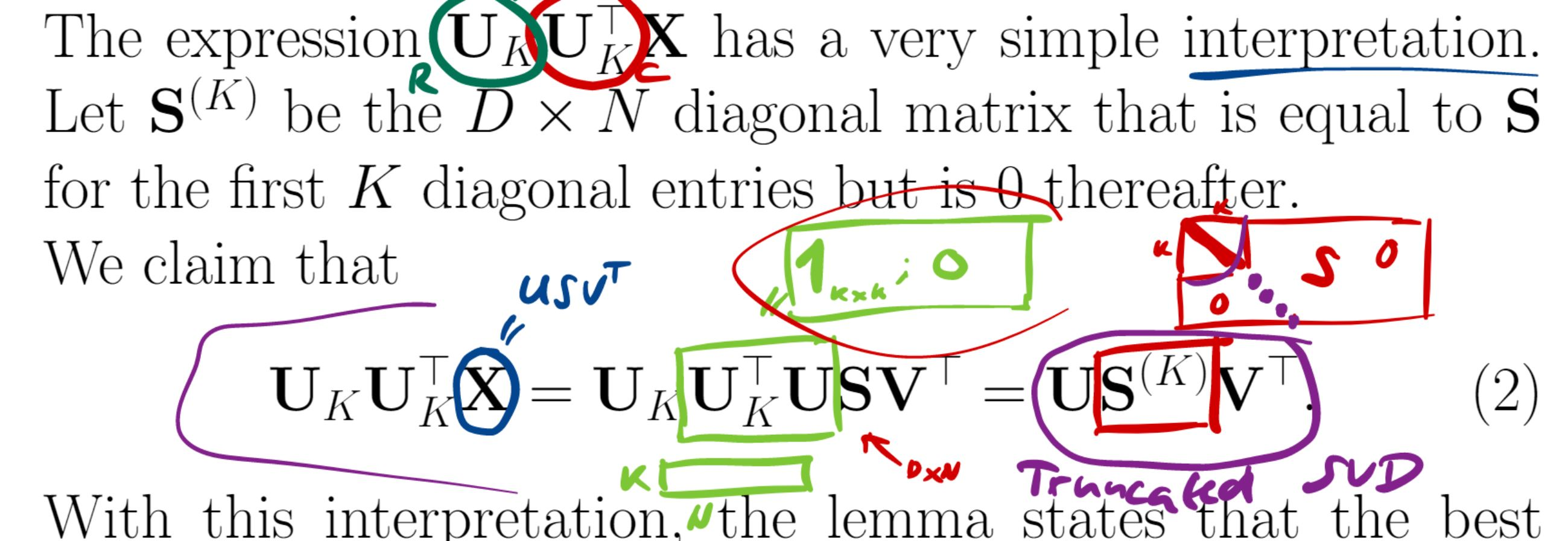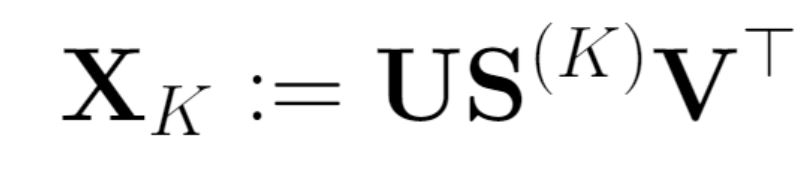### Truncated SVD

Hi!
I'm a little confused about the dimensions of the matrices.
1) The Uk matrix has size DK, then the Uk^TU matrix must have size KD and after multiplying with the S matrix, the resulting matrix has size KN. But the Sk matrix has size D*N... And how was the last equality obtained - the matrix Uk replaced by the matrix U?2) Also, these 2 formulas seem contradictory to me. They are the same except for U and Uk.3) Why multiplication by matrix U gives compression? Isn't Uk the compression matrix?Thank you in advance!

Page 1 of 1